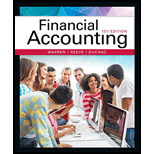# Boyd Co. produces and sells aviation equipment. On the first day of its fiscal year, Boyd Co. issued $80,000,000 of five-year, 9% bonds at a market (effective) interest rate of 12%, with interest payable semiannually. Compute the following, presenting figures used in your computations: a. The amount of cash proceeds from the sale of the bonds. Use the tables of present values in Exhibits 8 and 10. Round to the nearest dollar. b. The amount of discount to be amortized for the first semiannual interest payment period, using the interest method. Round to the nearest dollar. c. The amount of discount to be amortized for the second semiannual interest payment period, using the interest method. Round to the nearest dollar. d. The amount of the bond interest expense for the first year.BuyFindarrow_forward ### Financial Accounting 15th Edition Carl Warren + 2 others Publisher: Cengage Learning ISBN: 9781337272124 #### Solutions Chapter SectionBuyFindarrow_forward ### Financial Accounting 15th Edition Carl Warren + 2 others Publisher: Cengage Learning ISBN: 9781337272124 Chapter 14, Problem 26E Textbook Problem 73 views ## Boyd Co. produces and sells aviation equipment. On the first day of its fiscal year, Boyd Co. issued$80,000,000 of five-year, 9% bonds at a market (effective) interest rate of 12%, with interest payable semiannually. Compute the following, presenting figures used in your computations:        a.       The amount of cash proceeds from the sale of the bonds. Use the tables of present values in Exhibits 8 and 10. Round to the nearest dollar.       b.       The amount of discount to be amortized for the first semiannual interest payment period, using the interest method. Round to the nearest dollar.        c.       The amount of discount to be amortized for the second semiannual interest payment period, using the interest method. Round to the nearest dollar.       d.       The amount of the bond interest expense for the first year.

(a)

To determine

Calculate the amount of cash proceeds (present value) from the sale of the bonds.

### Explanation of Solution

Bonds Payable: Bonds payable are referred to long-term debts of the business, issued to various lenders known as bondholders, generally in multiples of $1,000 per bond, to raise fund for financing the operations. Discount on bonds payable: It occurs when the bonds are issued at a low price than the face value. Effective-interest method of amortization: It is an amortization model that apportions the amount of bond discount or premium based on the market interest rate. Present Value: The value of today’s amount expected to be paid or received in the future at a compound interest rate is called as present value. Determine the amount of cash proceeds (present value) from the sale of the bonds. Step 1: Calculate the semiannual interest on bonds. Interest=Face value×Face interest rate×Interest time period=$80,000,000×9%×612=$3,600,000 Step 2: Calculate the present value of interest.  Particulars Amount Interest payment (a)$3,600,000 PV factor at semiannual market interest rate of 6% for 10 periods (b) 7.36009 Present value (a)×(b) \$26,496,324

Table (1)

Note: The present value factor for 10 periods at 6% interest would be 7

(b)

To determine

Calculate the amount of discount to be amortized for the first semiannual interest payment period.

(c)

To determine

Calculate the amount of discount to be amortized for the second semiannual interest payment period.

(d)

To determine

Calculate the amount of bond interest expense for first year.

### Still sussing out bartleby?

Check out a sample textbook solution.

See a sample solution

#### The Solution to Your Study Problems

Bartleby provides explanations to thousands of textbook problems written by our experts, many with advanced degrees!

Get Started

Find more solutions based on key concepts
What is a loan amortization schedule, and what are some ways these schedules are used?

Fundamentals of Financial Management (MindTap Course List)

In what ways is economics a science?

Principles of Microeconomics (MindTap Course List)

Define commodity IT asset.

Accounting Information Systems

Why do options typically sell at prices higher than their exercise values?

Fundamentals of Financial Management (MindTap Course List)

Why do U.S. corporations build manufacturing plants abroad when they can build them at home?

Fundamentals of Financial Management, Concise Edition (with Thomson ONE - Business School Edition, 1 term (6 months) Printed Access Card) (MindTap Course List)